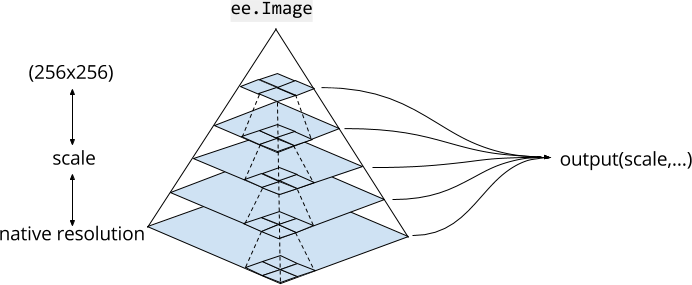# Scale

Understanding how Earth Engine handles scale is crucial to interpreting scientific results obtained from Earth Engine. Here, scale means pixel resolution. Unlike other GIS and image processing platforms, the scale of analysis is determined from the output, rather than the input. Specifically, when you make a request for results, an image to display or a statistic, for example, you specify the scale at which data is input to the analysis. This concept is illustrated in Figure 1.Figure 1. A graphic representation of an image dataset in Earth Engine. Dashed lines represent the pyramiding policy for aggregating 2x2 blocks of 4 pixels. Earth Engine uses the scale specified by the output to determine the appropriate level of the image pyramid to use as input.

## Image Pyramids

Image assets in Earth Engine exist at multiple scales, in image pyramids. The pyramiding policy (represented by dashed lines in Figure 1) determines how each pixel at a given level of the pyramid is computed from the aggregation of a 2x2 block of pixels at the next lower level. For continuous valued images, the pixel values of upper levels of the pyramid are the mean of pixels at the next lower level. For discrete valued images, pixel values of upper levels of the pyramid are a sample (usually the top left pixel) of pixels at the next lower level.

The lowest level of the image pyramid represents image data at native resolution, when it is ingested into Earth Engine. During ingestion, the data are aggregated (according to the pyramiding policy) to create higher pyramid levels. The data are aggregated until the entire image fits within a 256x256 pixel tile. When you use an image in your code, Earth Engine chooses a level of the pyramid with the closest scale less than or equal to the scale specified by your analysis and resamples (using nearest neighbor by default) as necessary.

## Scale of analysis

Scale of analysis in Earth Engine is determined on a "pull" basis. The scale at which to request inputs to a computation is determined from the output. For example, if you add an image to the map with `Map.addLayer()`, the zoom level of the map in the Code Editor determines the scale at which inputs are requested from the image pyramid. For other computations, you specify `scale` as an argument. For example, using the NIR band of a Landsat image, which has 30 meters native resolution:

### Code Editor (JavaScript)

```var image = ee.Image('LANDSAT/LC08/C01/T1/LC08_044034_20140318').select('B4');

var printAtScale = function(scale) {
print('Pixel value at '+scale+' meters scale',
image.reduceRegion({
reducer: ee.Reducer.first(),
geometry: image.geometry().centroid(),
// The scale determines the pyramid level from which to pull the input
scale: scale
}).get('B4'));
};

printAtScale(10); // 8883
printAtScale(30); // 8883
printAtScale(50); // 8337
printAtScale(70); // 9215
printAtScale(200); // 8775
printAtScale(500); // 8300```

In this example, note that the pixel value at a constant location (the image centroid) varies based on scale. This is due to the fact that different pyramid levels are selected for different scales. For similar scales, nearest neighbor resampling results in the same pixel value being returned. The important point is that by varying the scale, different image inputs are requested.

When you visualize an image by adding it to the map, Earth Engine determines scale from the zoom level. Consider the following simple example, which simply displays a Landsat image:

### Code Editor (JavaScript)

```var image = ee.Image('LANDSAT/LC08/C01/T1/LC08_044034_20140318');
Map.centerObject(image, 17);
Map.addLayer(image, {bands: ['B4', 'B3', 'B2'], max: 12000}, 'image');```

The map starts zoomed all the way in, such that the native resolution pixels are clearly visible. Zooming out far enough will not display the same pixels, but will instead display higher levels of the image pyramid. It is also worth noting that the Code Editor map uses the maps mercator (EPSG:3857) projection, so the appropriate level of the image pyramid also needs to be reprojected prior to display. Learn more about how Earth Engine handles projections from the projections doc.

[]
[]